# 0708-1300/fact

(diff) ← Older revision | Latest revision (diff) | Newer revision → (diff)
If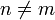$n\neq m$ then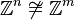$\mathbb{Z}^n\not\cong\mathbb{Z}^m$.
Assume that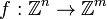$f:\mathbb{Z}^n\rightarrow\mathbb{Z}^m$ is an isomorphism. Let$A$ be the matrix of$f$ in the canonical basis and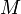$M$ the maximum of the absolute values of the entries of$A$. If we evaluate$f$ in all the vectors of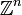$\mathbb{Z}^n$ who's entries have absolute values less than or equal to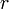$r$ (there are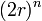$(2r)^n$ of such elements) then we get elements who's entries have absolute value less than or equal to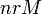$nrM$ (there are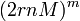$(2rnM)^m$ of such elements in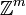$\mathbb{Z}^m$). Since$f$ is injective we must have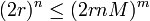$(2r)^n\leq(2rnM)^m$ for every$r$. Replacing$f$ by its inverse if necessary we can assume that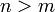$n>m$ but if this is the case the inequality above can not be true for arbitrarily large values of$r$.